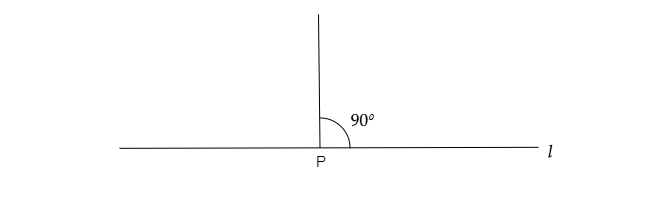# Draw line $l$. Take any point $P$ on the line. Using a set square, draw a line perpendicular to line $l$ at the point $P$.

To do: To draw line $l$ and take any point $P$ on the line. By using a set square, draw a line perpendicular to line $l$ at the point $P$.

Solution:

1.  Draw a line $l$.

2.  Take a point $P$. and using set square we draw an angle of $90^o$.